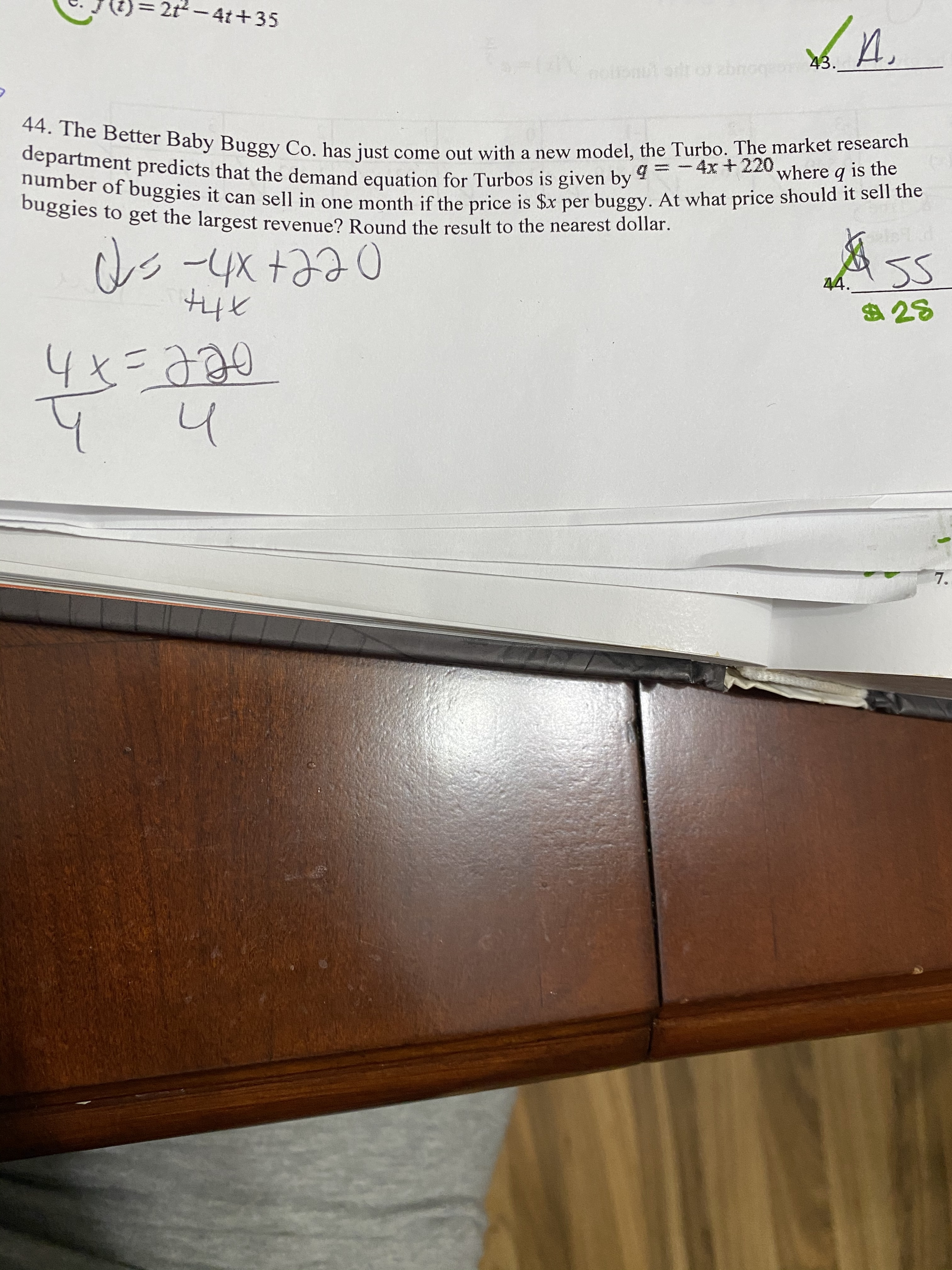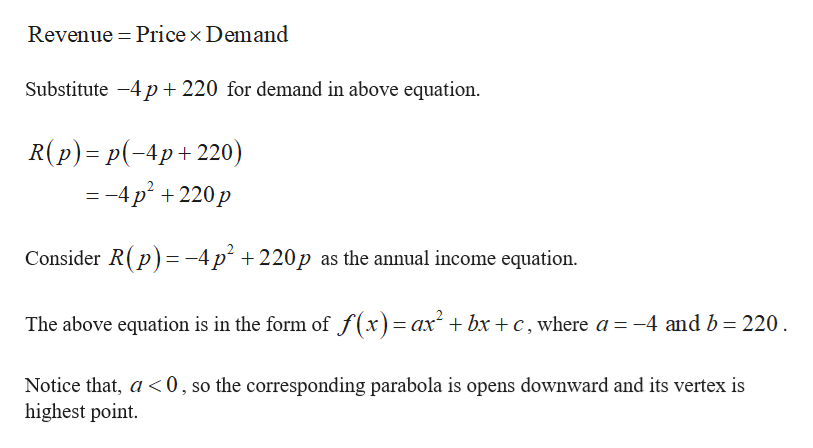# 2t- 4t+35И.nottonut ont o zbnogeo43.44. The Better Baby Buggy Co. has just come out with a new model, the Turbo. The market researchdepartment predicts that the demand equation for Turbos is given by =- 4x + 220 where q is thenumber of buggies it can sell in one month if the price is \$x per buggy. At what price should it sell thebuggies to get the largest revenue? Round the result to the nearest dollar.AsS44.\$284x=770हैeे07.

Question
11 views

This homework problem is part of Math Optimization class.help_outlineImage Transcriptionclose2t- 4t+35 И. nottonut ont o zbnogeo43. 44. The Better Baby Buggy Co. has just come out with a new model, the Turbo. The market research department predicts that the demand equation for Turbos is given by =- 4x + 220 where q is the number of buggies it can sell in one month if the price is \$x per buggy. At what price should it sell the buggies to get the largest revenue? Round the result to the nearest dollar. AsS 44. \$28 4x=770 हैeे0 7. fullscreen
check_circle

star
star
star
star
star
1 Rating
Step 1

The provided demand equation is given by

Step 2

The relationship between revenu...help_outlineImage TranscriptioncloseRevenue = Price × Demand Substitute -4 p+ 220 for demand in above equation. R(p) = p(-4p+220) = -4 p² + 220 p Consider R(p) = -4 p² + 220p as the annual income equation. The above equation is in the form of f(x)= ax + bx + c, where a =-4 and b= 220. %3D Notice that, a <0, so the corresponding parabola is opens downward and its vertex is highest point. fullscreen

### Want to see the full answer?

See Solution

#### Want to see this answer and more?

Solutions are written by subject experts who are available 24/7. Questions are typically answered within 1 hour.*

See Solution
*Response times may vary by subject and question.
Tagged in

### Other